# How to Calculate and Solve for Fired Linear Shrinkage | Refractories

The fired linear shrinkage is illustrated by the image below.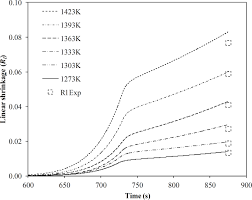To compute for fired linear shrinkage, two essential parameters are needed and these parameters are Dried Length (DL) and Fired Length (FL).

The formula for calculating fired linear shrinkage:

FLS = 100(DL – FL/DL)

Where:

FLS = Fired Linear Shrinkage
DL = Dried Length
FL = Fired Length

Given an example;
Find the fired linear shrinkage when the dried length is 32 and the fired length is 12.

This implies that;

DL = Dried Length = 32
FL = Fired Length = 12

FLS = 100(DL – FL/DL)
FLS = 100((32 – 12)/32)
FLS = 100(20/32)
FLS = 100(0.625)
FLS = 62.5

Therefore, the fired linear shrinkage is 62.5%.

Nickzom Calculator – The Calculator Encyclopedia is capable of calculating the fired linear shrinkage.

To get the answer and workings of the fired linear shrinkage using the Nickzom Calculator – The Calculator Encyclopedia. First, you need to obtain the app.

You can get this app via any of these means:

You can also try the demo version via https://www.nickzom.org/calculator

Apple (Paid) – https://itunes.apple.com/us/app/nickzom-calculator/id1331162702?mt=8
Once, you have obtained the calculator encyclopedia app, proceed to the Calculator Map, then click on Materials and Metallurgical under Engineering.Now, Click on Refractories under Materials and Metallurgical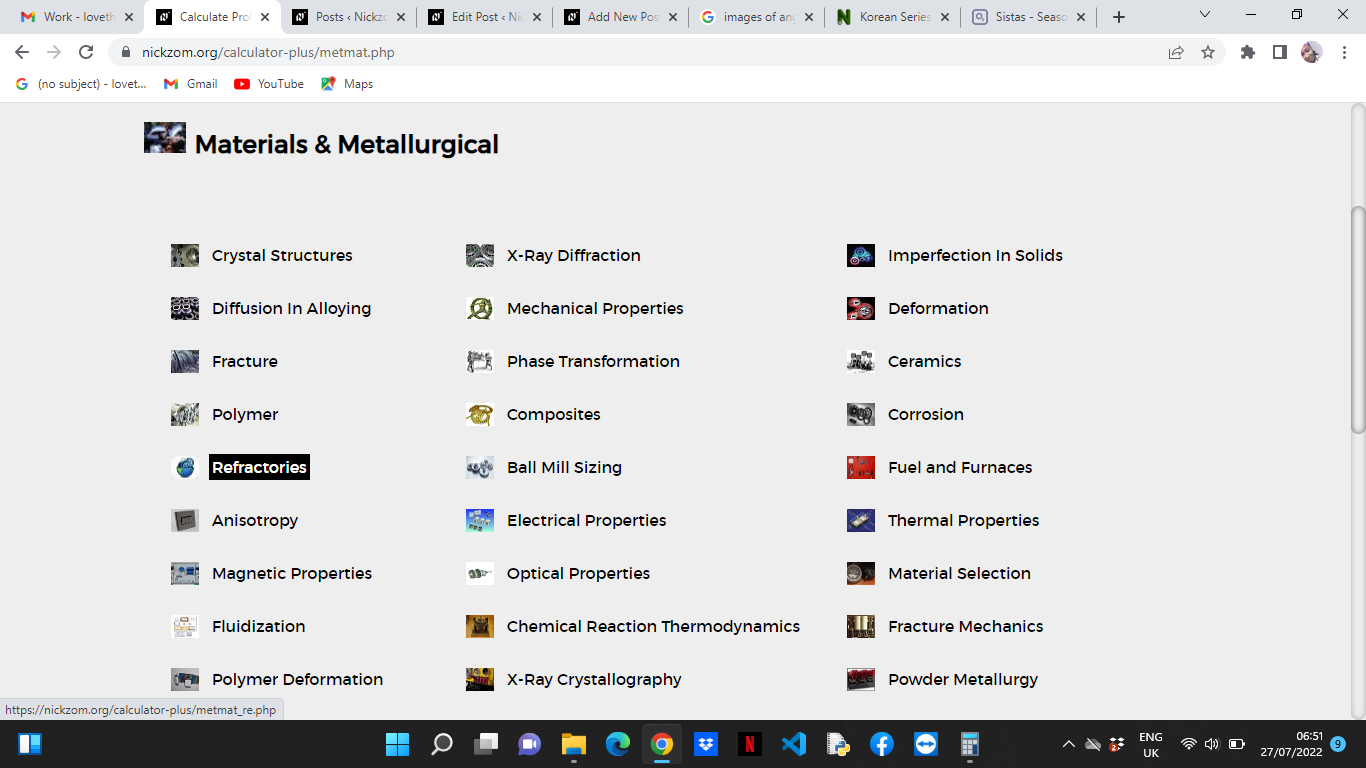Now, Click on Fired Linear Shrinkage under Refractories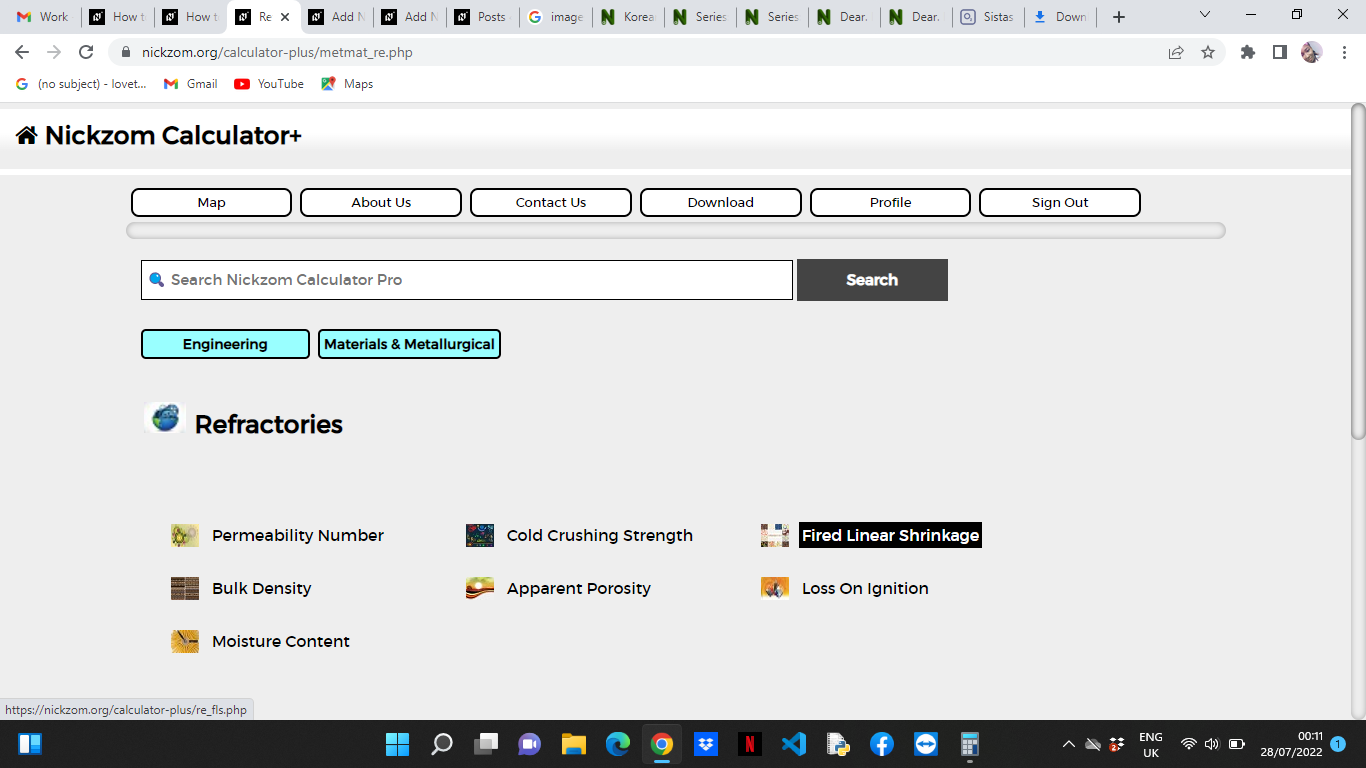The screenshot below displays the page or activity to enter your values, to get the answer for the fired linear shrinkage according to the respective parameters which are the Dried Length (DL) and Fired Length (FL).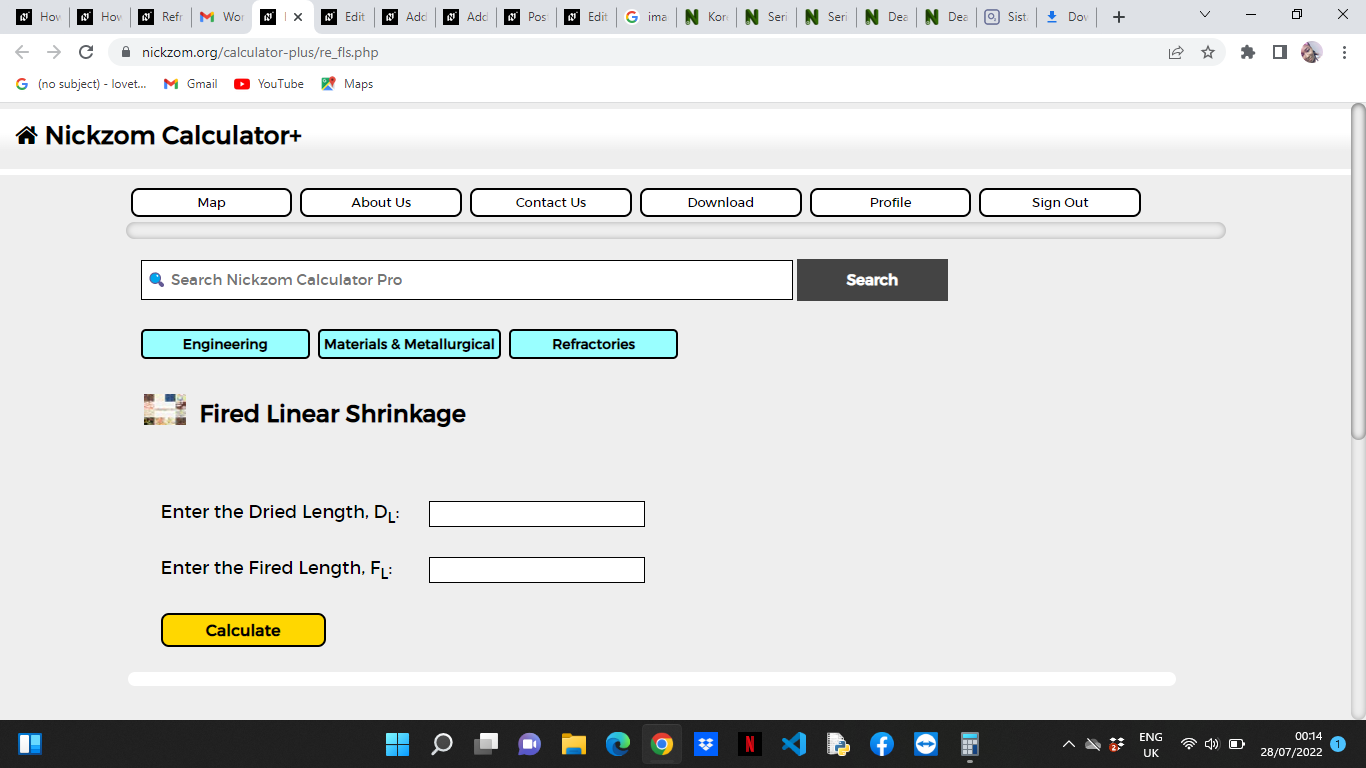Now, enter the values appropriately and accordingly for the parameters as required by the Dried Length (DLis 32 and Fired Length (FL) is 12.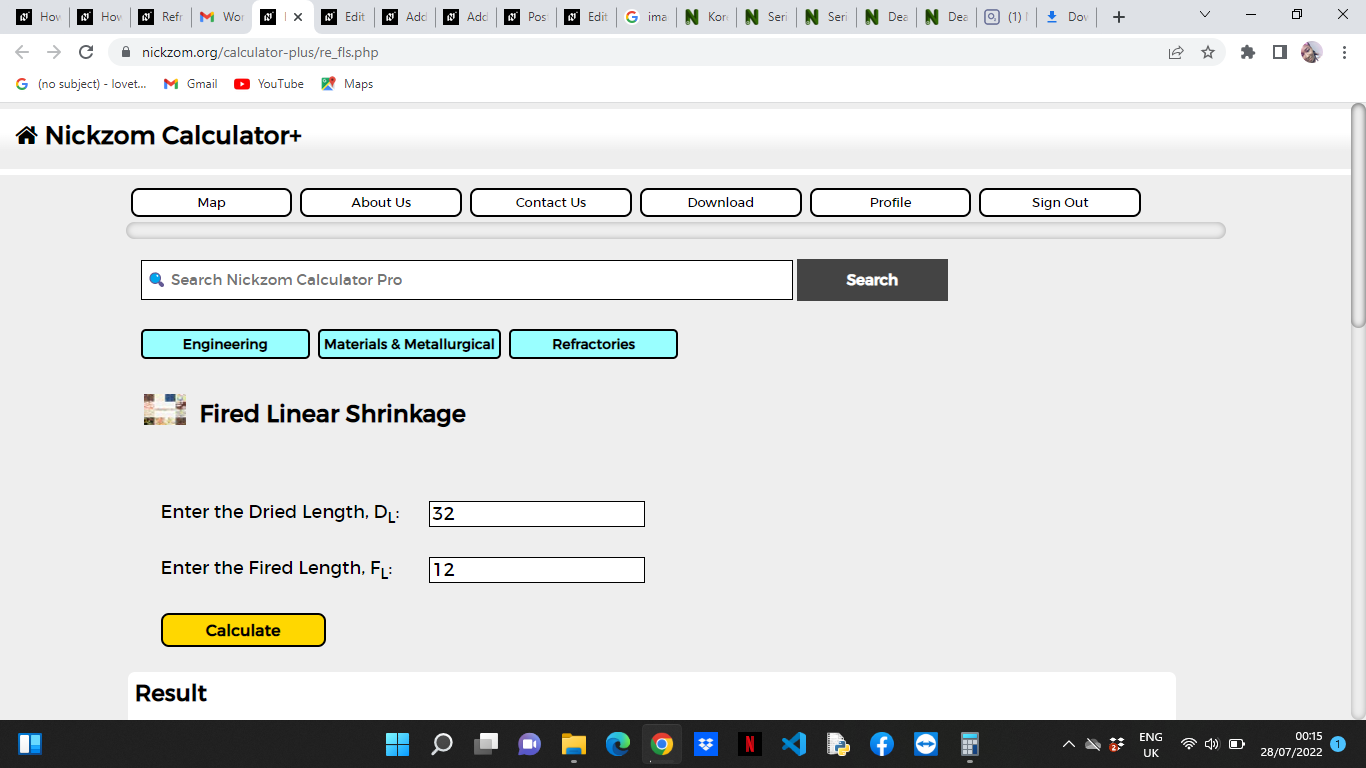Finally, Click on Calculate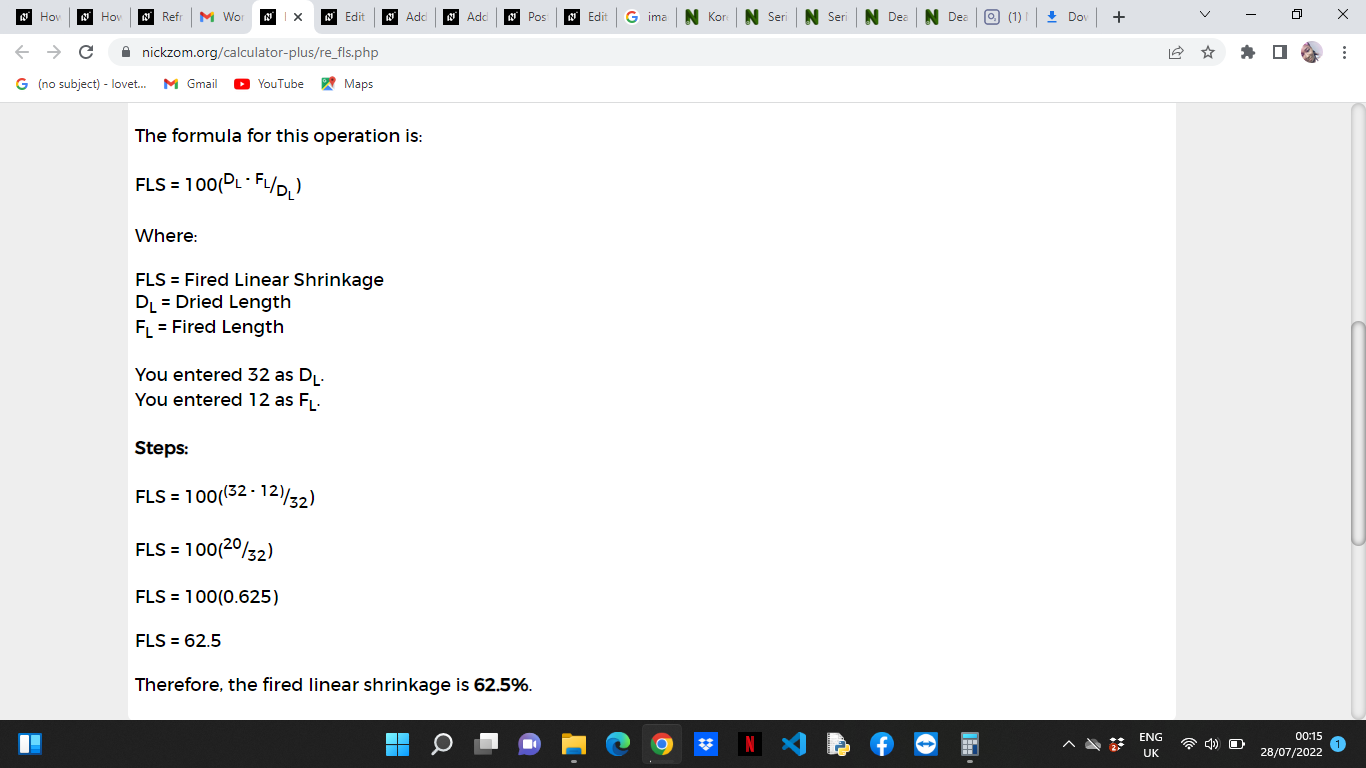As you can see from the screenshot above, Nickzom Calculator– The Calculator Encyclopedia solves for the fired linear shrinkage and presents the formula, workings and steps too.